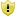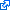Data Scientist

# News

## Statistical testing of hypothesis streams and cascades

Statistical hypothesis testing was developed in an age when calculations were performed by hand and individual hypotheses were considered in isolation.

In the modern information era, it is often desirable to assess large numbers of related hypotheses. Unless explicit steps are taken to control the risk, it becomes a near certainty that if large numbers of the hypotheses that one seeks to reject are in fact true then many will be rejected in error. Multiple testing corrections control this risk, but previous approaches have required that the hypotheses to be assessed (or at least an upper bound on their number) are known in advance.

Sometimes, however, there is a need to test some hypotheses before other related hypotheses are known.  We call this a hypothesis stream. In some hypothesis streams, the decision of which hypotheses to reject at one time affect which hypotheses will be considered subsequently. We call this a hypothesis cascade.

One context in which hypothesis cascades arise is model selection, such as forward feature selection.  This process starts with an empty model.  Then, at each step all models are considered that add a single feature to the current model. A hypothesis test is conducted on every such new model.  The model with the best test result (lowest p) is then accepted, and the process is repeated considering all single feature additions to this new model. This is repeated until no improvement is statistically significant.  The model selected at each round determines the models to be tested subsequently, and it is not possible to determine in advance how many rounds it will take until the process terminates and hence how many statistical tests will be performed.

We have developed a statistical testing procedure that strictly controls the risk of familywise error – the risk of incorrectly rejecting any null hypothesis out of the entire stream. It does so without requiring any information other than the history of the analysis so far and the current pool of hypotheses under consideration. Studies of its performance in the context of selecting the edges to include in a graphical model indicate that it achieves this strict control while maintaining high statistical power.

Statistical hypothesis testing provides a powerful and mature toolkit for data analytics. These techniques help bring these powerful tools to bear in the age of big data.

Watch the promotional video for our paper:

[embedyt] http://www.youtube.com/watch?v=FBlhhebFhTI&width=640&height=390&rel=0&showinfo=0[/embedyt]

Publication

Webb, G. I., & Petitjean, F.
Proceedings of the ACM SIGKDD Conference on Knowledge Discovery and Data Mining, KDD-16, pp. 1255-1264, 2016.Top reviewer score (4.75/5.0), shortlisted for best paper award and invited to ACM TKDE journal KDD-16 special issue[Bibtex] [Abstract]  → Related papers and software

``````@InProceedings{WebbPetitjean16,
Title = {A multiple test correction for streams and cascades of statistical hypothesis tests},
Author = {Webb, Geoffrey I and Petitjean, Francois},
Booktitle = {Proceedings of the ACM SIGKDD Conference on Knowledge Discovery and Data Mining, KDD-16},
Year = {2016},
Pages = {1255-1264},
Publisher = {ACM Press},
Abstract = {Statistical hypothesis testing is a popular and powerful tool for inferring knowledge from data. For every such test performed, there is always a non-zero probability of making a false discovery, i.e.~rejecting a null hypothesis in error. Familywise error rate (FWER) is the probability of making at least one false discovery during an inference process. The expected FWER grows exponentially with the number of hypothesis tests that are performed, almost guaranteeing that an error will be committed if the number of tests is big enough and the risk is not managed; a problem known as the multiple testing problem. State-of-the-art methods for controlling FWER in multiple comparison settings require that the set of hypotheses be pre-determined. This greatly hinders statistical testing for many modern applications of statistical inference, such as model selection, because neither the set of hypotheses that will be tested, nor even the number of hypotheses, can be known in advance.
This paper introduces Subfamilywise Multiple Testing, a multiple-testing correction that can be used in applications for which there are repeated pools of null hypotheses from each of which a single null hypothesis is to be rejected and neither the specific hypotheses nor their number are known until the final rejection decision is completed.
To demonstrate the importance and relevance of this work to current machine learning problems, we further refine the theory to the problem of model selection and show how to use Subfamilywise Multiple Testing for learning graphical models.
We assess its ability to discover graphical models on more than 7,000 datasets, studying the ability of Subfamilywise Multiple Testing to outperform the state of the art on data with varying size and dimensionality, as well as with varying density and power of the present correlations. Subfamilywise Multiple Testing provides a significant improvement in statistical efficiency, often requiring only half as much data to discover the same model, while strictly controlling FWER.},
Comment = {Top reviewer score (4.75/5.0), shortlisted for best paper award and invited to ACM TKDE journal KDD-16 special issue},
Doi = {10.1145/2939672.2939775},
Keywords = {Association Rule Discovery and statistically sound discovery and scalable graphical models and Learning from large datasets and DP140100087},
Related = {statistically-sound-association-discovery},
Url = {http://dl.acm.org/authorize?N19100}
}``````
``ABSTRACT Statistical hypothesis testing is a popular and powerful tool for inferring knowledge from data. For every such test performed, there is always a non-zero probability of making a false discovery, i.e.~rejecting a null hypothesis in error. Familywise error rate (FWER) is the probability of making at least one false discovery during an inference process. The expected FWER grows exponentially with the number of hypothesis tests that are performed, almost guaranteeing that an error will be committed if the number of tests is big enough and the risk is not managed; a problem known as the multiple testing problem. State-of-the-art methods for controlling FWER in multiple comparison settings require that the set of hypotheses be pre-determined. This greatly hinders statistical testing for many modern applications of statistical inference, such as model selection, because neither the set of hypotheses that will be tested, nor even the number of hypotheses, can be known in advance. This paper introduces Subfamilywise Multiple Testing, a multiple-testing correction that can be used in applications for which there are repeated pools of null hypotheses from each of which a single null hypothesis is to be rejected and neither the specific hypotheses nor their number are known until the final rejection decision is completed. To demonstrate the importance and relevance of this work to current machine learning problems, we further refine the theory to the problem of model selection and show how to use Subfamilywise Multiple Testing for learning graphical models. We assess its ability to discover graphical models on more than 7,000 datasets, studying the ability of Subfamilywise Multiple Testing to outperform the state of the art on data with varying size and dimensionality, as well as with varying density and power of the present correlations. Subfamilywise Multiple Testing provides a significant improvement in statistical efficiency, often requiring only half as much data to discover the same model, while strictly controlling FWER.``Data Scientist